# 資料視覺化之美-以matlab為工具

Matlab視覺化基礎（見下圖，該圖源自一位中科大的博士），裡面涉及到畫圖的一些基礎內容，其github連結，文末會提供原始碼下載：\ https://github.com/Pjer-zhang/matlabPlotCheatsheet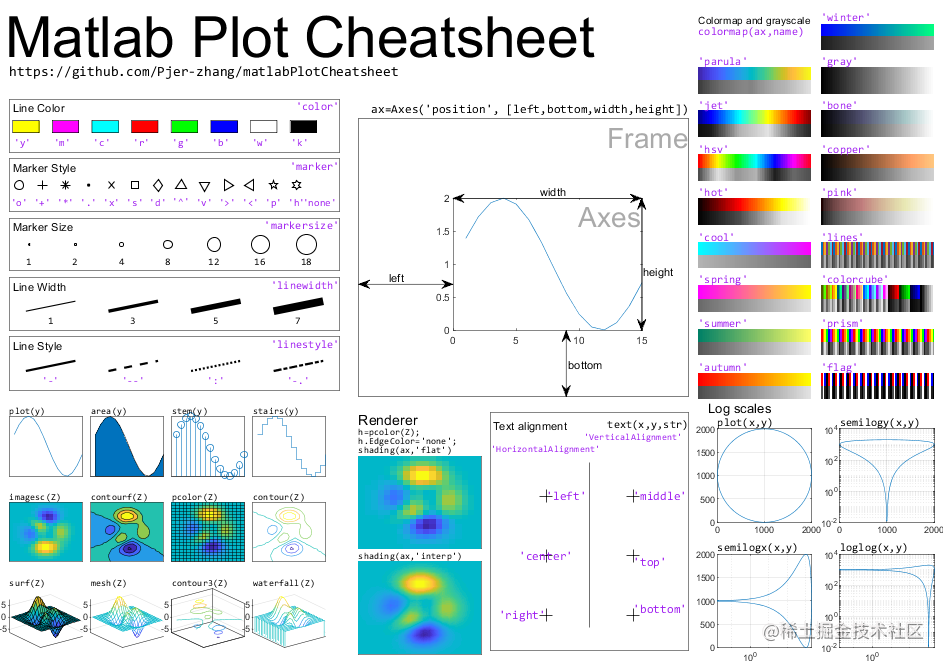## 資料視覺化作品（Matlab版本，含程式碼）

### 1、 三維切片圖：通過切片觀測三維資料的變化

#### 1.1 簡單示例

```matlab [X,Y,Z] = meshgrid(-2:.2:2); V = X.*exp(-X.^2-Y.^2-Z.^2);

xslice = [-1.2,0.8,2];
yslice = []; zslice = 0; slice(X,Y,Z,V,xslice,yslice,zslice) ```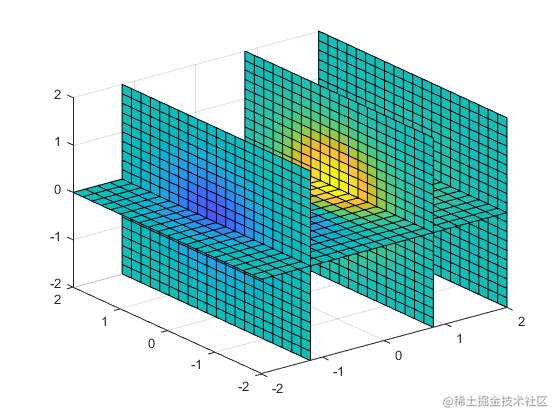#### 1.2 稍複雜的示例

```matlab 1. [x,y,z,v] = flow; %%flow()函式可以生成幾個三維向量 2. x1 = min(min(min(x)));x2 = max(max(max(x))); %此處x為255025的矩陣。 3. y1 = min(min(min(x)));y2 = max(max(max(x))); 4. z1 = min(min(min(x)));y2 = max(max(max(x))); 5. sx = linspace(x1+1.5,x2,5); 6. sy = 0; 7. sz = 0; 8. slice(x,y,z,v,sx,sy,sz); 9. view([-38,38]);shading interp; 10. colormap jet; 11. 12. set(gcf,'color',[1 1 1])

```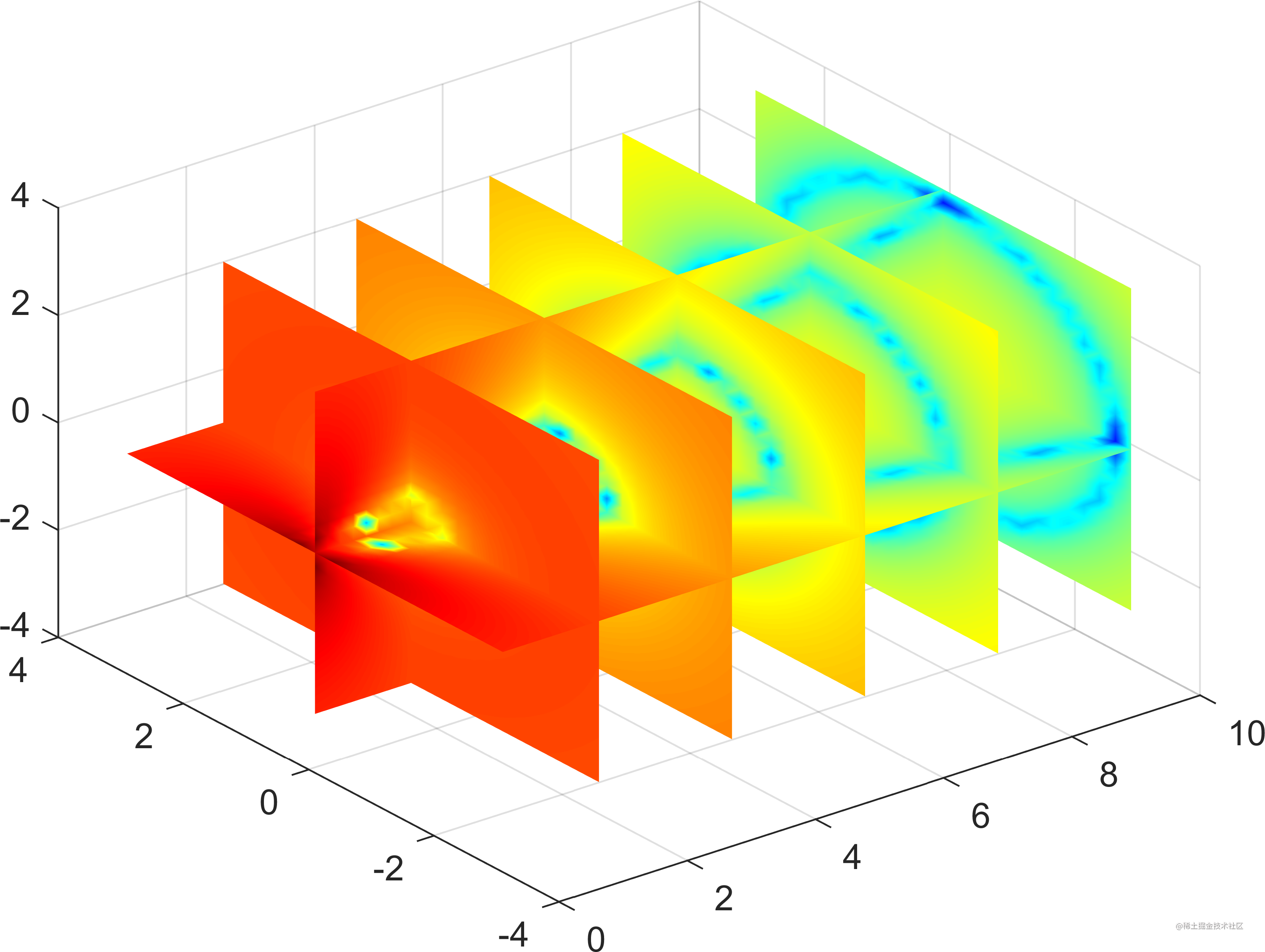#### 1.3 現實的案例：空氣汙染物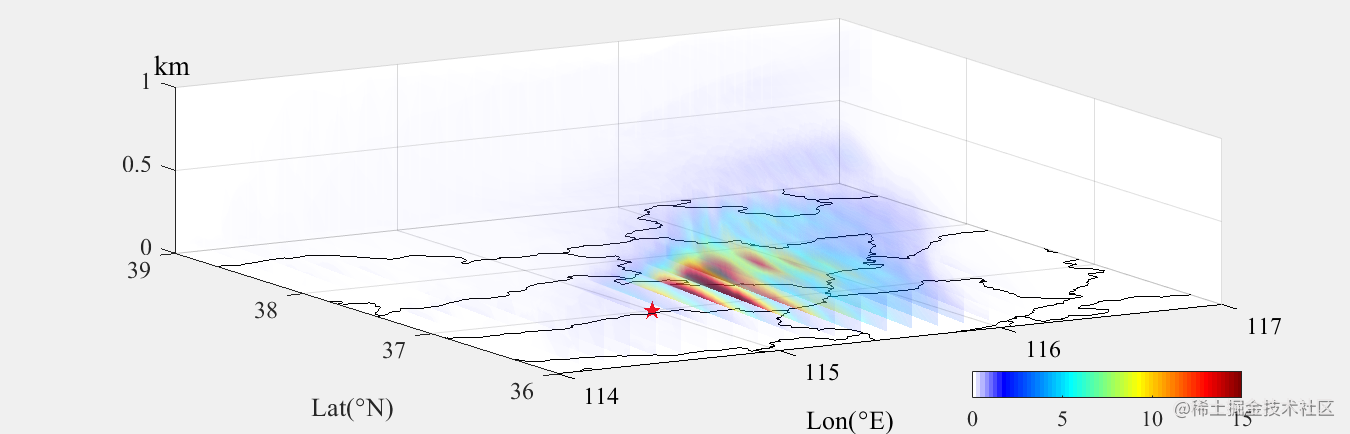### 2、三維流場

2.
3. % Calculate homogenous turbulence values at each (x,y,z)
4. u = sin(pix).cos(piy).cos(piz);
5. v = -cos(pi
x).sin(piy).cos(piz);
6. w = sqrt(2/3)cos(pix).cos(piy).sin(piz);
7.
8. % Draw a 3 dimensional quiver plot using the quiver3 function
9. figure
10. quiver3(x, y, z, u, v, z)
11.
12. % Set the axis limits
13. axis([-1 1 -1 1 -1 1])
14.
15. % Add title and axis labels
16. title('Turbulence Values')
17. xlabel('x')
18. ylabel('x')
19. zlabel('z')

```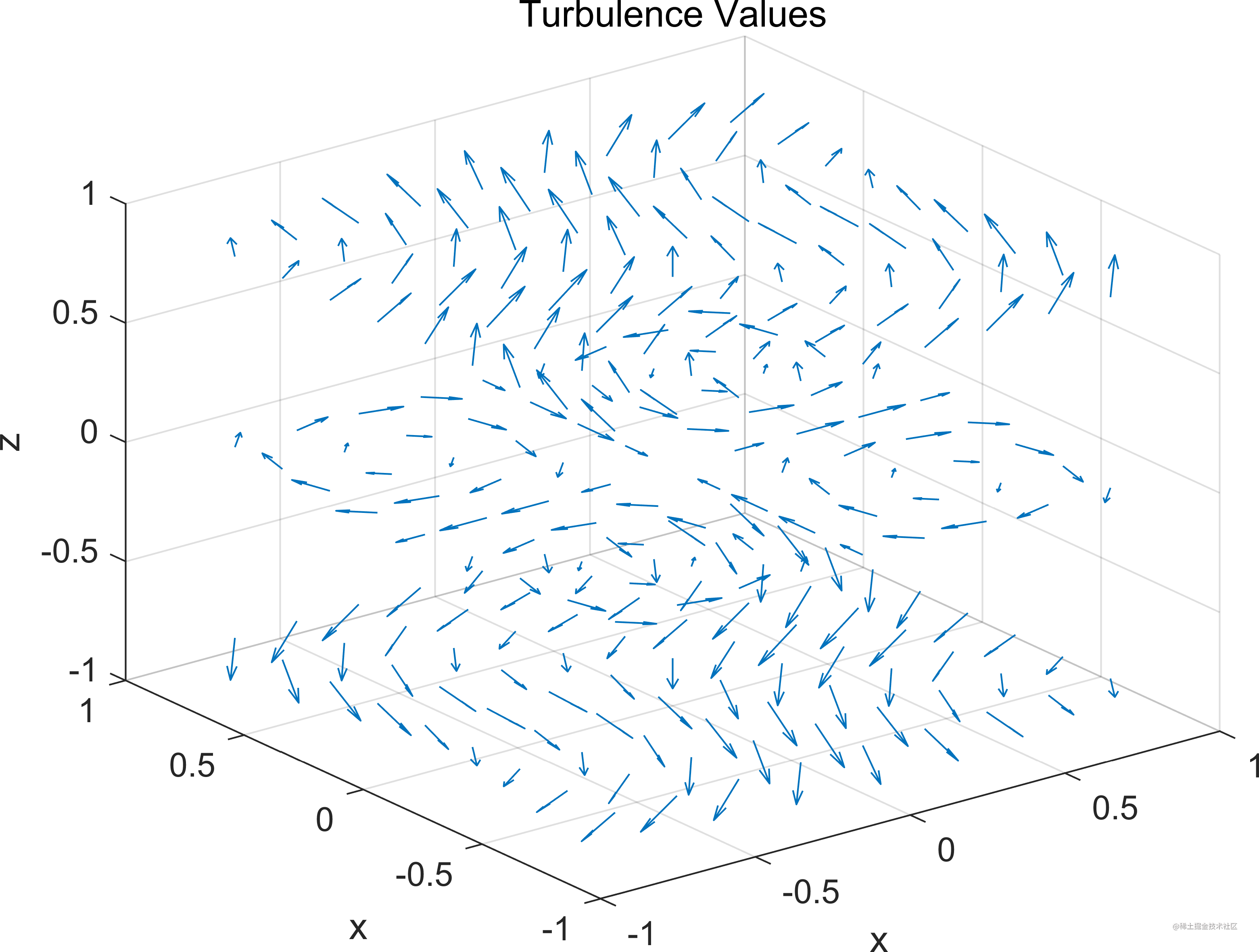### 3、流場的渦量切片

```matlab 1. close all
3.
4. mycmp=[[ones(20,1),(0.05:0.05:1)',(0.05:0.05:1)'];[(1:-0.05:0.05)',(1:-0.05:0.05)',ones(20,1)]];
5. cav = curl(x,y,z,u,v,w); %計算旋度
6. h = slice(x,y,z,cav,[90 134],59,0); %切片
8. daspect([1 1 1]);
9. axis tight
10. colormap(mycmp);
11. caxis([-5,5])
12. camlight
13. set([h(1),h(2)],'ambientstrength',.6);

```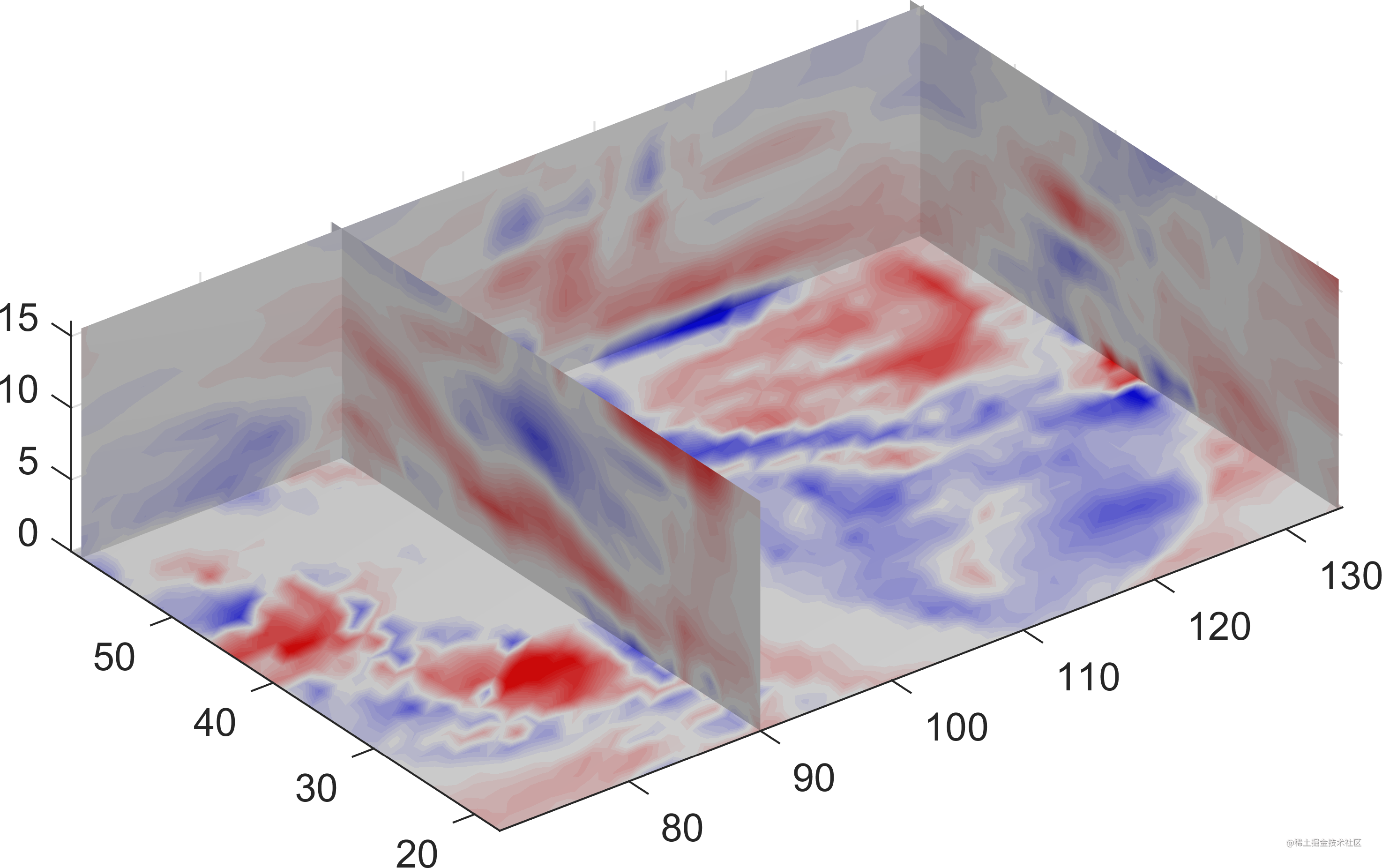### 4、運動的流場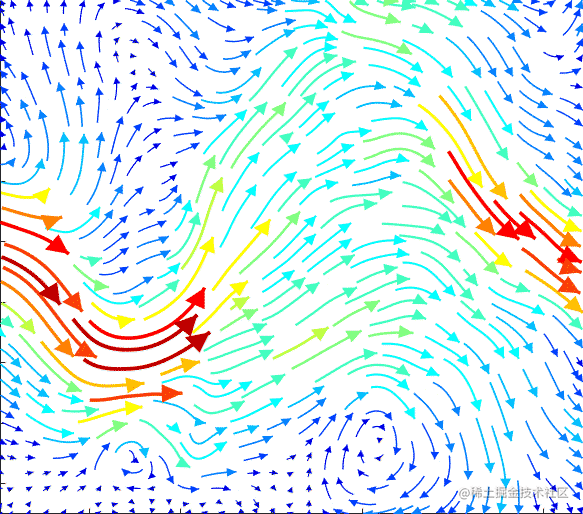### 5、三維流帶圖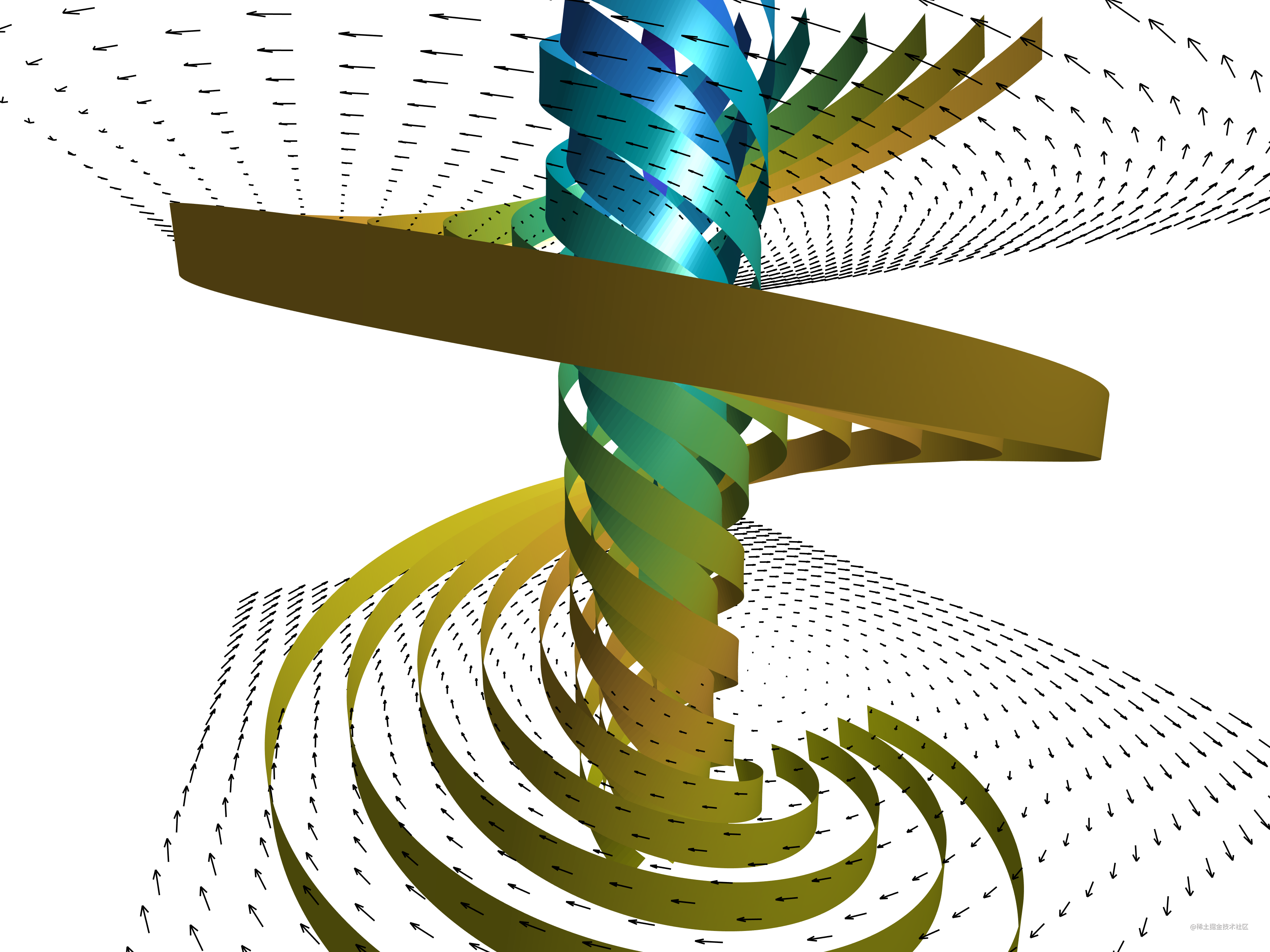### 6、流管圖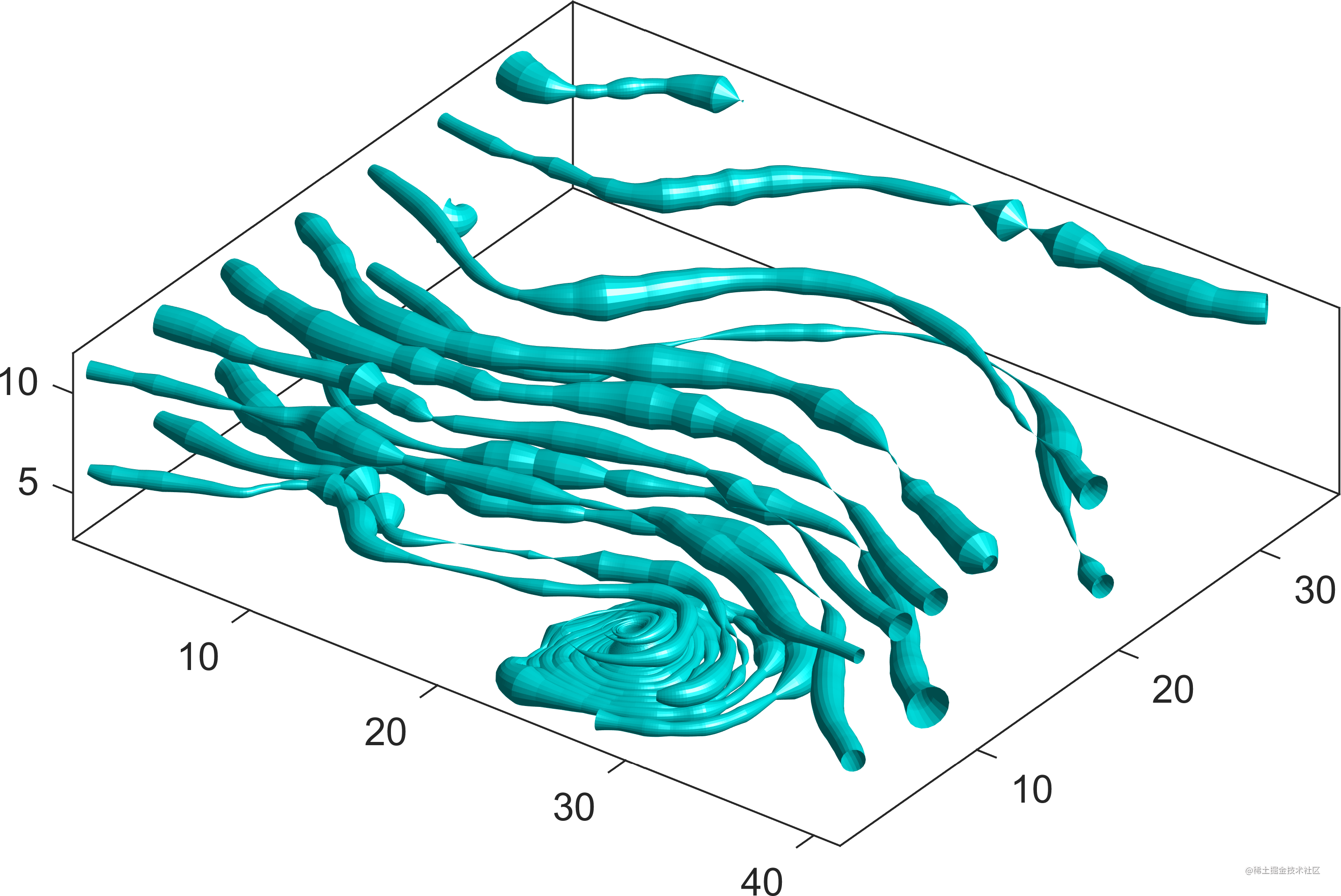### 7、三維速度場全貌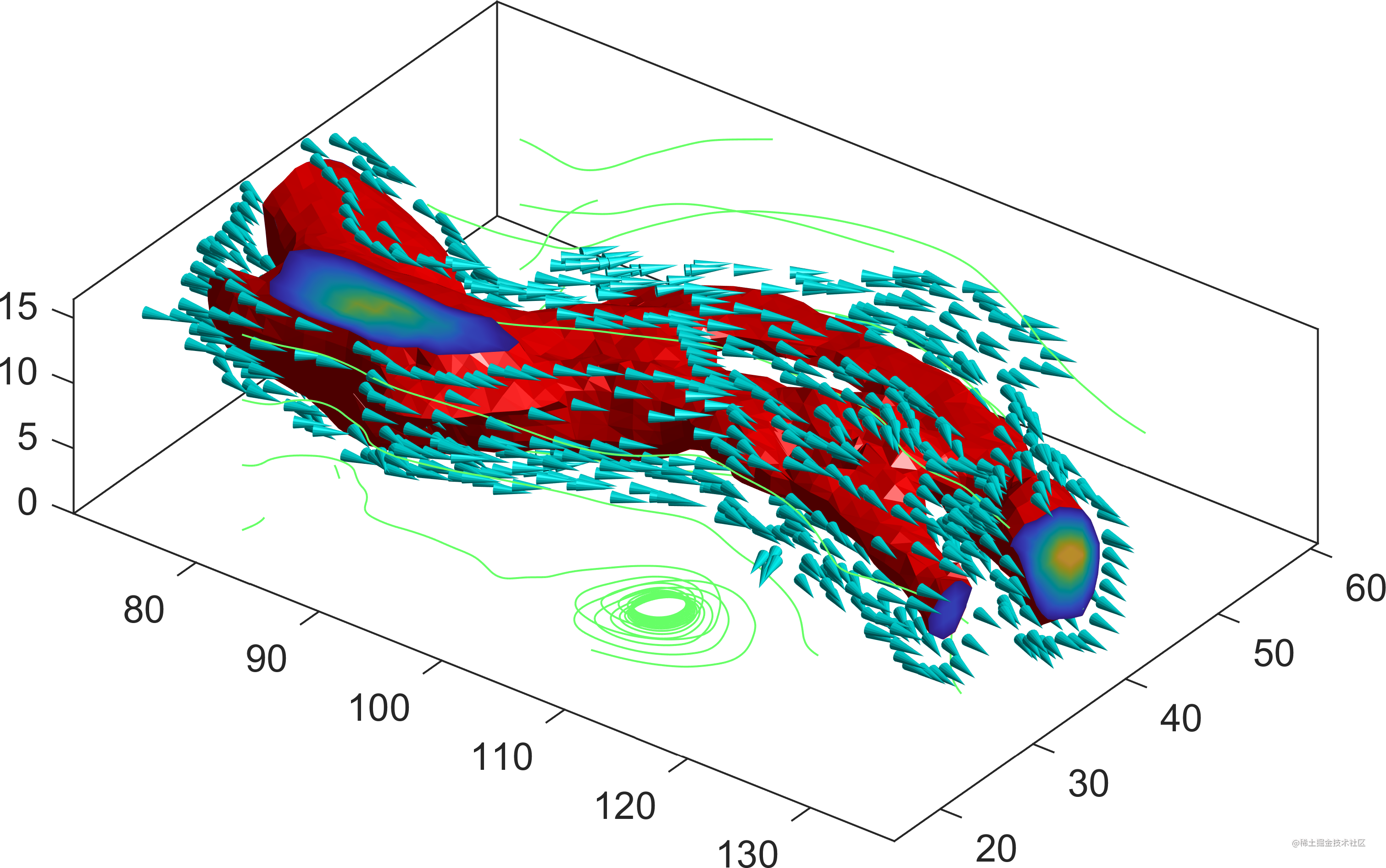### 8、運動的地球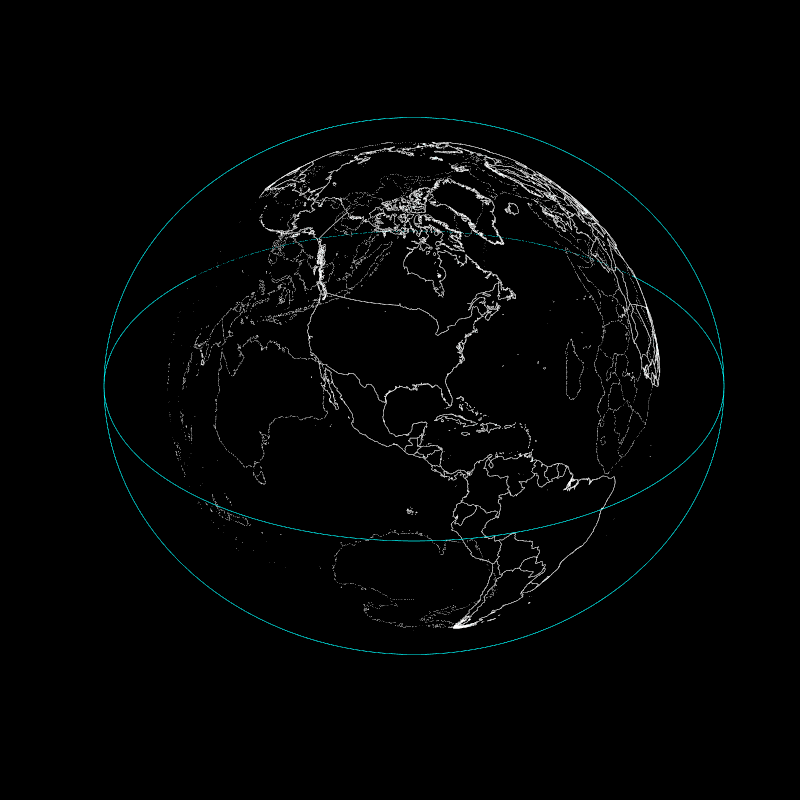「其他文章」